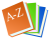German to Scottish Gaelic dictionary

 Look up:This German to Scottish Gaelic dictionary searches words in both directions at the same time.
Both German to Scottish Gaelic and Scottish Gaelic to German translations will be listed at once.

 Apfel (m) ubhal (m)Baby (n); Säugling (m); Kleinkind (n) leanabh (m), leanaban (m), naoidhean (m), pàisde (m)Baum (m); Baum craobh (f)Blume (f) dìthean (m); flùr (m)Bruder (m) bràthair (m)Buch (n) leabhar (m)danke; danke schön; vielen Dank tapadh leat; tapadh leibh; taingFeuer (n) teine (m)Frau (f) bean (f); boireannach (m) Freund (m); Freundin (f) caraid (m); bancharaid (f)Frieden (m); Friede (m); Scheinfriede (m) sìth (f)Frucht (f) meas (m), toradh (m)Geld (n) airgead (m); airgiod (m)hallo; guten Tag; servus; grüß Gott halò; latha math; hòighHaus (n); Häuser taigh (m)hören; zuhören èisd; èistHund (m); Rüde (m); Hündin (f) cù (m), madadh (m)Junge (m); Knabe (m); Bub (m) balach (m); balachan (m); gille (m)Katze (f); Kater (m); Kätzin (f) cat (m)lesen leugh Liebe (f) rùn (m)Mädchen (n) caileag (f); nighean (f); nighneag Mann (m); Herr (m) fear (m); duine (m)Mutter (f) màthair (f)Nahrung (f); Essen (n); Lebensmittel (n) biadh (m)Pferd (n); Ross (n); Pferdehengst (m); Pferdestute (f) eachSchule (f) sgoil (f)Schwester (f) piuthar (f)Stadt (f) baile mòr (m), dùn (m)Tag (m) latha (m)Tier (n) beathach (m), ainmhidh (m)Vater (m) athair (m)Zeit (f) àm (m)
Scottish Gaelic to German dictionary